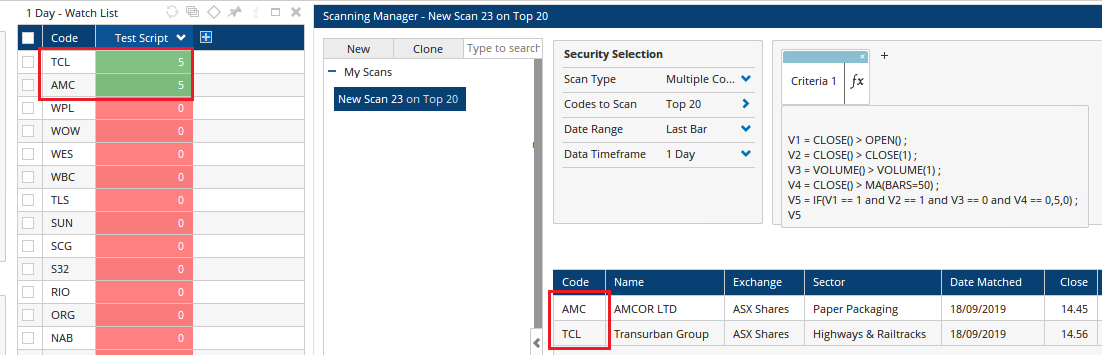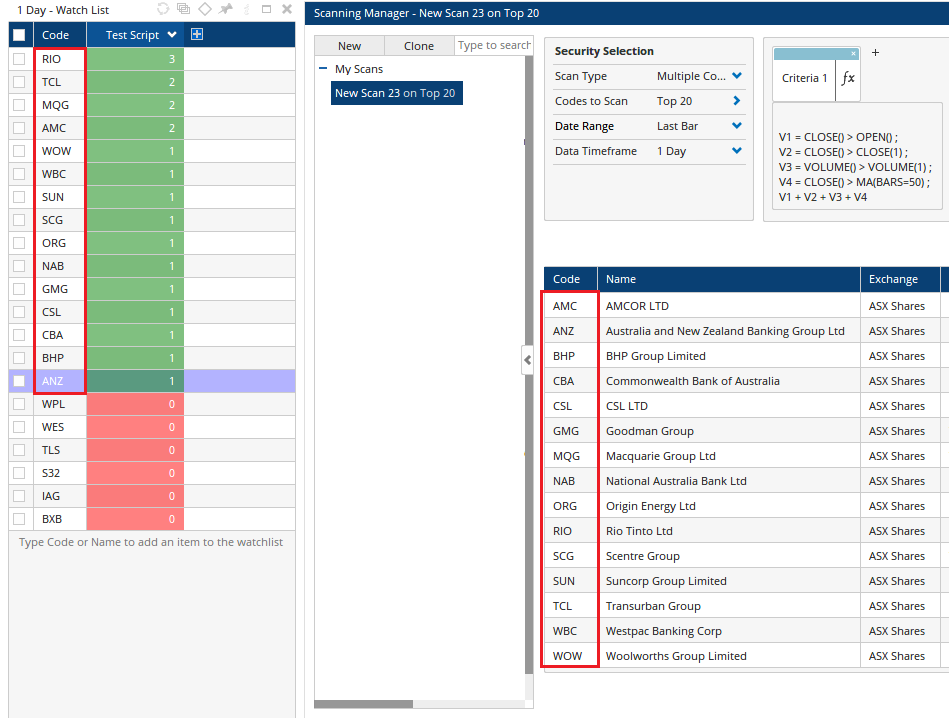# Scans with non Boolean Scripts

Author: Optuma Team Last updated: Sep 19, 2019 14:02

## Overview

In the majority of cases the Optuma scanning manager requires a script which produces a Boolean result (1 for True, 0 for False). However from Optuma version 1.5 and later there are some specific instances where this is no longer required.

### Example 1

In cases where an IF(),or multiple IF functions have been setup to produce values greater than 1.

V1 = CLOSE() > OPEN() ;
V2 = CLOSE() > CLOSE(1) ;
V3 = VOLUME() > VOLUME(1) ;
V4 = CLOSE() > MA(BARS=50) ;
V5 = IF(V1 == 1 and V2 == 1 and V3 == 0 and V4 == 0,5,0) ;
V5

The above script produces a value of 5 when the criteria passes. In Optuma v1.5 and later this same script will produce a pass result in a scan.### Example 2

This same principle applies for cases where multiple Boolean variables are added up to produce a custom rank value.

For example:

V1 = CLOSE() > OPEN() ;
V2 = CLOSE() > CLOSE(1) ;
V3 = VOLUME() > VOLUME(1) ;
V4 = CLOSE() > MA(BARS=50) ;
V1 + V2 + V3 + V4